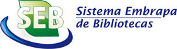Autor
 Palavra-chave
 Tipo do documento
 Ano
 País
 Idioma
 Ordenar por: Relevância Autor Título AnoPrimeira ... 1 ... ÚltimaFlores,José H. N.; Rettore Neto,Osvaldo; Faria,Lessandro C.; Timm,Luís C.. ABSTRACT: The objective of this study was to determine the variability of the head loss as a function of the emitter geometry as well as to develop a relation between local head loss caused by the emitter insertion and geometric characteristics of the emitter pipe, using index of obstruction for dripper pipes with non-coaxial emitters. For this, an experimental bench was developed to control the system and obtain the variables pertinent to the study. From the value of the total head loss in the emitter pipe and the value obtained with calculation of the distributed head loss in the pipe, the difference of these values was local head loss caused by the insertion of the emitter. Total head loss in the emitter pipe and local head loss on the emitter presented... Tipo: Info:eu-repo/semantics/article Palavras-chave: Non-coaxial emitters; Obstruction index; Trickle irrigation; Head loss. Ano: 2017 URL: http://www.scielo.br/scielo.php?script=sci_arttext&pid=S0100-69162017000601091Rocha,Hermes S. da; Marques,Patricia A. A.; Camargo,Antonio P. de; Frizzone,José A.; Saretta,Ezequiel. ABSTRACT Assuming that a roughness meter can be successfully employed to measure the roughness on the internal surface of irrigation pipes, this research had the purpose of defining parameters and procedures required to represent the internal surface roughness of plastic pipes used in irrigation. In 2013, the roughness parameter Ra, traditional for the representation of surface irregularities in most situations, and the parameters Rc, Rq, and Ry were estimated based on 350 samples of polyvinyl chloride (PVC) and low-density polyethylene (LDPE) pipes. Pressure losses were determined from experiments carried out in laboratory. Estimations of pressure loss varied significantly according to the roughness parameters (Ra, Rc, Rq, and Ry) and the corresponding... Tipo: Info:eu-repo/semantics/article Palavras-chave: Friction factor; Head loss; Roughness meter. Ano: 2017 URL: http://www.scielo.br/scielo.php?script=sci_arttext&pid=S1415-43662017000300143Pimenta,Bruna D.; Robaina,Adroaldo D.; Peiter,Marcia X.; Mezzomo,Wellington; Kirchner,Jardel H.; Ben,Luis H. B.. ABSTRACT One of the parameters involved in the design of pressurized hydraulic systems is the pressure drop in the pipes. The verification of the pressure drop can be performed through the Darcy-Weisbach formulation, which considers a coefficient of head loss (f) that can be estimated by the implicit Colebrook-White equation. However, for this determination, it is necessary to use numerical methods or the Moody diagram. Because of this, numerous explicit approaches have been proposed to overcome such limitation. In this sense, the objective of this study was to analyze the explicit approximations of the f for pressurized conduits in comparison to the Colebrook-White formulation, determining the most precise ones so that they can be used as an alternative... Tipo: Info:eu-repo/semantics/article Palavras-chave: Head loss; Darcy-Weisbach; Turbulent flow regime; Performance index. Ano: 2018 URL: http://www.scielo.br/scielo.php?script=sci_arttext&pid=S1415-43662018000500301Minhoni,Renata T. de A.; Pereira,Francisca F. S.; Silva,Tatiane B. G. da; Castro,Evanize R.; Saad,João C. C.. ABSTRACT The Darcy-Weisbach equation is the most recommended equation for determining the pressure loss in pressurized pipes because of its wide applicability. However, one of the largest obstacles to implementing this equation is the friction factor (f) calculation. This factor can be precisely determined using the Colebrook equation, which is implicit. Thus, the objective of this study was to compare six explicit equations for calculating the Darcy-Weisbach friction factor with the implicit Colebrook equation based on the relative error. Based on the results, the equations of Vatankhah and Offor & Alabi were the most highly recommended. These six explicit formulas showed a mean relative error of less than ± 1% compared to the Colebrook equation,... Tipo: Info:eu-repo/semantics/article Palavras-chave: Absolute roughness; Colebrook equation; Forced conduits; Head loss; Hydraulic load; Reynolds number. Ano: 2020 URL: http://www.scielo.br/scielo.php?script=sci_arttext&pid=S0100-69162020000200258